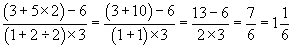What Are Exponents?

# ?

Key Terms

o         Exponent

o         Base

o         Order of operations

Objectives

o         Recognize and convert between repeated multiplication and exponential representations

o         Evaluate simple exponents

o         Use the order of operations to properly evaluate multiple operations in a single expression

Multiplication is a way to represent repeated addition. For instance, instead of adding 5 + 5 + 5 + 5 + 5 + 5, we can simply multiply 5 by 6. Similarly, we can also use an alternative representation of repeated multiplication. For instance, writing out the expression 10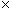101010 is rather cumbersome (especially if we needed to multiply 10 a few more times!). Instead, we can write this repeated multiplication using an exponent, which is the number of repeated factors. Exponents are written as a superscript number to the right of the number being multiplied. The number being multiplied (or "exponentiated") is called the base. Thus, for example,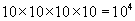Again, note that the exponent is the number of factors in the multiplication; thus, an exponent of nine would mean that the multiplication involves nine factors of the base. Below are some additional simple examples of exponents.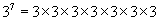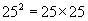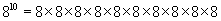The Language of Exponents

Perhaps more than any other basic math operation, exponents can be spoken of in a wide variety of ways. Although you may not use each of these expressions, the ability to recognize them is helpful. Below is a list of some of the different ways ab can be expressed in English. (Once again, the letters are used here simply as placeholders for unspecified numbers. The math expression ab could be 25, 73, or any other combination of base and exponent numbers. Regardless of their specific values, however, a is the base and b the exponent.)

"a raised to the power of b"

"a raised to the bth power"

"a raised to the b (or bth)"

"a to the b (or bth)"

In the case where b = 2, the expression "a squared" is often used, as is "a cubed" in the case of b = 3. In some cases, the same mathematician or scientist may even use different expressions to refer to the same exponent, all depending on the context and on the need for brevity or clarity.

On the basis of what we have seen so far, dealing with integer exponents greater than or equal to two is simple enough. But what about, for instance, exponents of zero or one? In the case of a base a raised to the power of one, the result is simply a. Consider the progression shown below.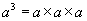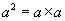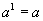Perhaps the case that is most unclear is that of a raised to the power of zero. Following the above pattern, the number of factors of a would be zero. In each case, we can multiply the result by one without changing anything. Using this context, we can show that a to the zero is simply one.

Interested in learning more? Why not take an online Pre-Algebra course?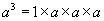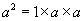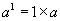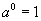Note that the value of a is not specified-thus, any number raised to the power of zero is one. Other interesting cases include negative exponents and fractional exponents.

Practice Problem: Evaluate each of the exponential expressions below.

a.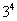b.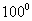c.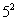d.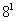Solution: The exponent is the number of factors of the base. In the case of the exponent being equal to zero, the answer is one, and in the case of the exponent being one, the answer is the base value.

a.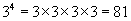b.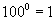c.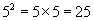d.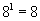Practice Problem: Write each of the following expressions in exponential form.

a.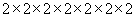b.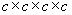c. 7     d.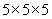Solution: The base of the exponential representation is the number that is being multiplied, and the exponent is the number of factors. Part b uses a letter (c) to represent an unspecified number; this letter can be treated just as a number would, however.

a.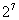b.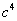c.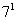d.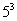Order of Operations

Before continuing with our study of exponents, we must consider the order of operations, which allows us to correctly interpret a series of mathematical operations written in a single expression. Consider, for instance, the following expression.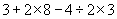The order in which we perform the listed operations will determine the answer: not every ordering of the operations yields the same result! We can illustrate this by taking two different approaches: first, we'll perform the operations in the order they are listed, and second, we'll perform the multiplication and division operations (in the listed order) followed by the addition and subtraction operations (in their listed order).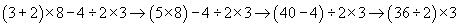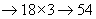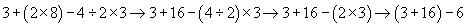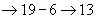Obviously, the two results are drastically different. Thus, an order of operations has been defined as a convention that we can use to properly interpret and express multiple mathematical operations. The order of operations is given below.

1.Parentheses: perform any operations or series of operations in parentheses

2.Exponents: evaluate any exponents

3.Multiplication/Division: perform multiplication and division operations in the order shown

(One way to remember this order of operations is by remembering the mnemonic word PEMDAS.) The first step is to evaluate all expressions enclosed in parentheses (or any other type of bracket, such as [ ] or { }). Because an expression within a set of parentheses may itself contain multiple operations or even additional parentheses, you must also evaluate that expression according to the order of operations (this may require that you use the first step multiple times). The second step is to evaluate any exponents. Next, perform multiplication and division operations in the order listed (from left to right). Finally, perform any addition and subtraction operations (in any order). Let's consider an example.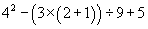First, we must evaluate the expression in the outer parentheses: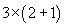Note that this expression contains another expression in parentheses; therefore, to properly evaluate it, we must once again apply the first step of the order of operations.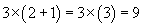Returning to the original expression, we must now evaluate exponents.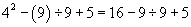Next, we look for multiplication and division operations. In this case, the expression contains only one.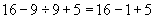We can now perform the remaining addition and subtraction operations in any order.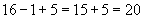Practice Problem: Evaluate each of the following expressions.

a.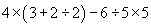b.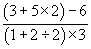Solution: Carefully follow the order of operations for each case (remember the word PEMDAS, if this helps you). Note in part b that the expressions in the numerator and denominator can be evaluated separately; although a fraction is a way of representing division, it is division of the entire numerator by the entire denominator.

a.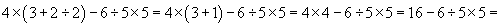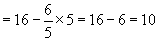b.